# 5:52 .11 LTE . a webassign.net Use a software program or a graphing utility to find the transition matrix from B to B", find the transition matrix from B' to B, venify that the tw...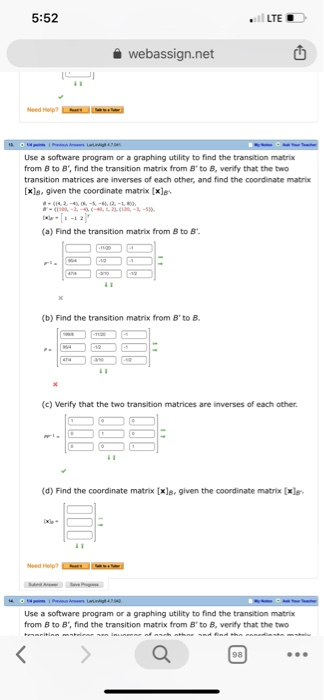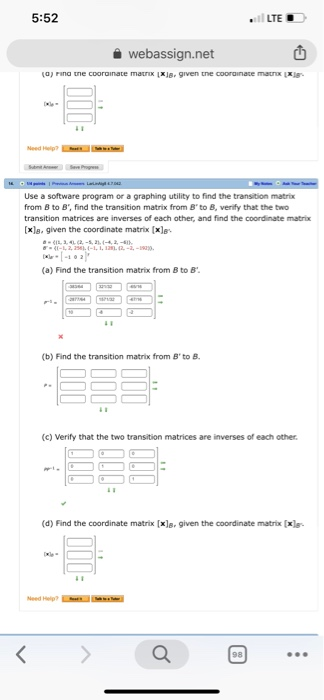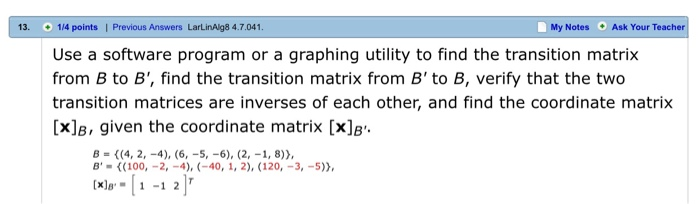5:52 .11 LTE . a webassign.net Use a software program or a graphing utility to find the transition matrix from B to B", find the transition matrix from B' to B, venify that the two transition matrices are inverses of each other, and find the coordinate matrix xls. given the coordinate matrix (xs (a) Find the transition matrix from B to B (b) Find the transition matrix from B' to B (c) Verify that the two transition matrices are inverses of each other (d) Find the coordinate matrix [xls. given the coordinate matrix [xle- Use a software program or a graphing utility to find the transition matrix from B to B", find the transition matrix from B' to B, venity that the two
5:52 LTED webassign.net a rina tne cooroinate maxxl given the coorainate matrx xe Use a software program or a graphing utility to find the transition matrix from B to B', find the transition matrix from B'to B, venify that the two transition matrices are inverses of each other, and find the coordinate matrix [xls. given the coordinate matrix [xs (a) Find the transition matrix from B to B (b) Find the transition matrix from B' to B. (c) Verify that the two transition matrices are inverses of each other (d) Find the coordinate matrix [xls. given the coordinate matrix [xa- a 回
5:52 .11 LTE . a webassign.net Use a software program or a graphing utility to find the transition matrix from B to B", find the transition matrix from B' to B, venify that the two transition matrices are inverses of each other, and find the coordinate matrix xls. given the coordinate matrix (xs (a) Find the transition matrix from B to B (b) Find the transition matrix from B' to B (c) Verify that the two transition matrices are inverses of each other (d) Find the coordinate matrix [xls. given the coordinate matrix [xle- Use a software program or a graphing utility to find the transition matrix from B to B", find the transition matrix from B' to B, venity that the two
13. 1/4 points I Previous Answers LarLinAlg8 4.7.041 My Notes Ask Your Teacher Use a software program or a graphing utility to find the transition matrix from B to B', find the transition matrix from B' to B, verify that the two transition matrices are inverses of each other, and find the coordinate matrix [x]B, given the coordinate matrix [x]B B-((100,-2,-4),-40, 1, 2),(120,-3,-5) (xl 1 -1 2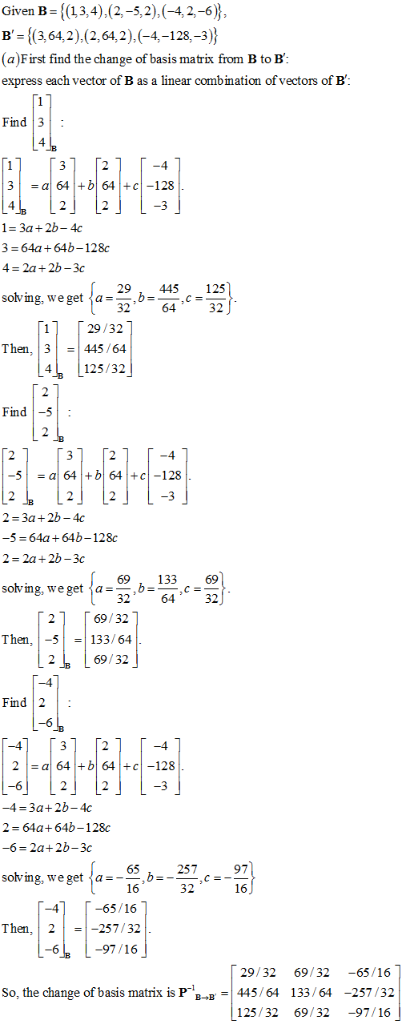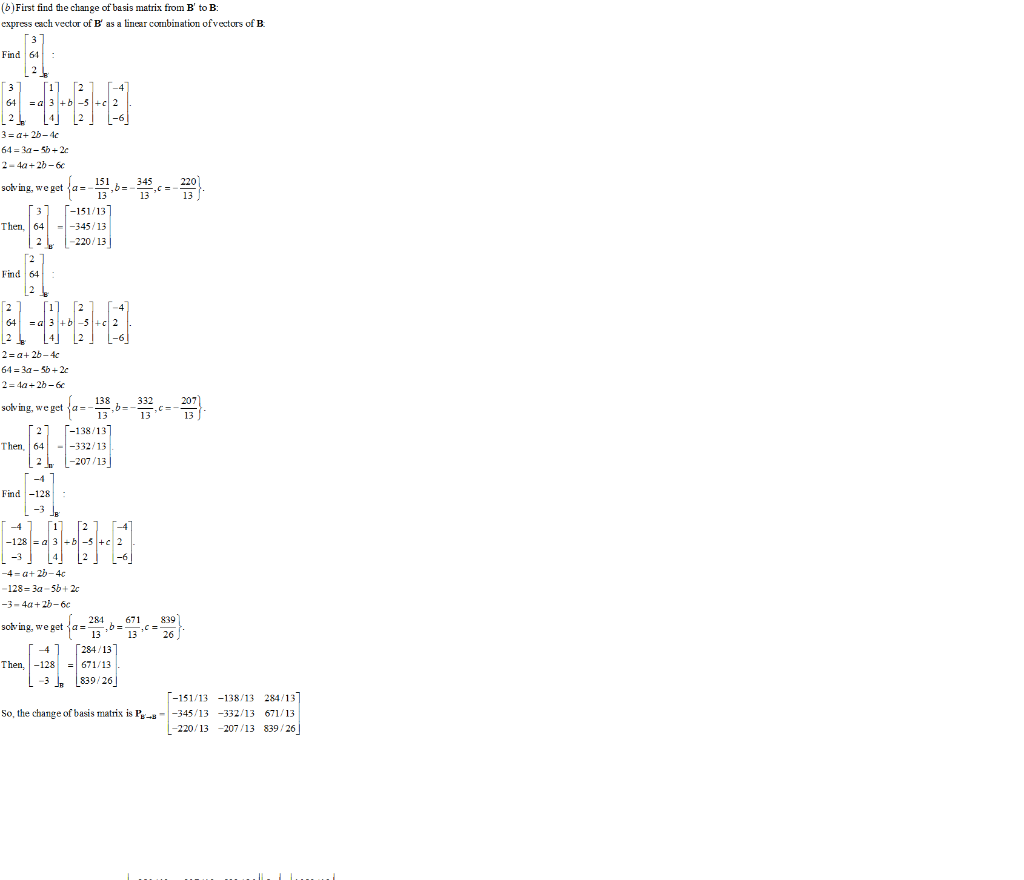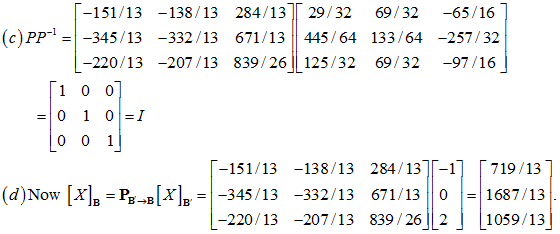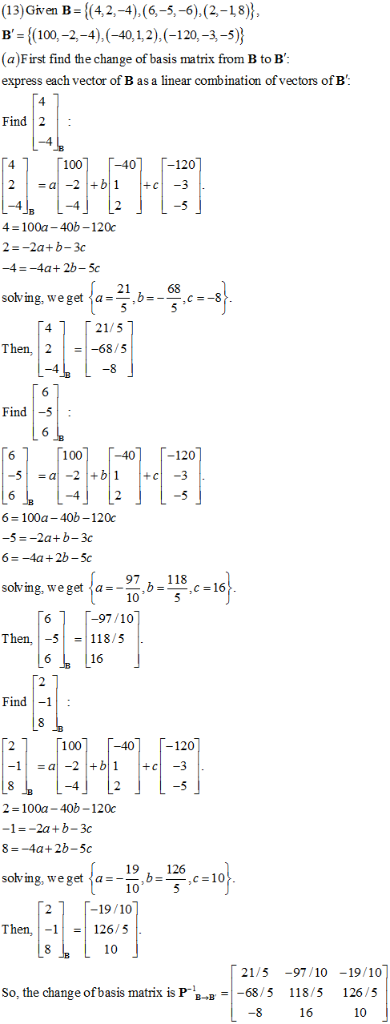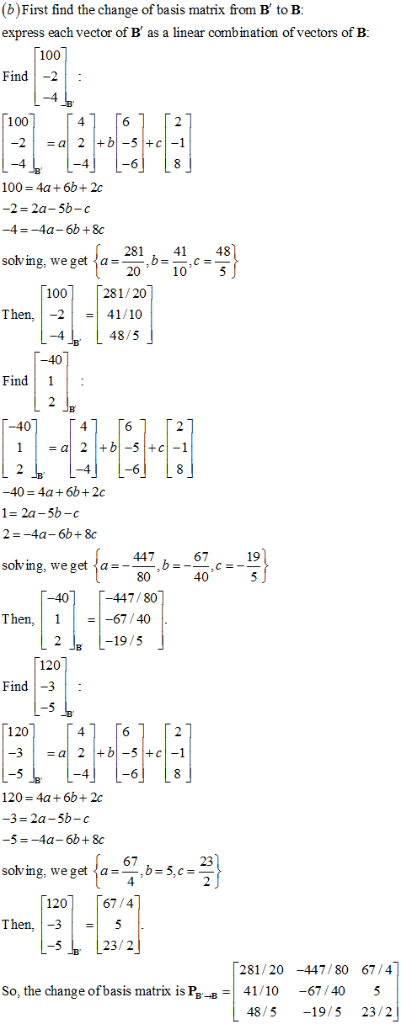##### Add Answer of: 5:52 .11 LTE . a webassign.net Use a software program or a graphing utility to find the transition matrix from B to B", find the transition matrix from B' to B, venify that the tw...
More Homework Help Questions Additional questions in this topic.